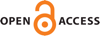Download this articleFor screen For printingRecent IssuesThe Journal About the Journal Editorial Board Editorial Interests Subscriptions Submission Guidelines Submission Page Policies for Authors Ethics Statement ISSN (electronic): 1472-2739 ISSN (print): 1472-2747 Author Index To Appear Other MSP Journals${\rm SL}_2$ quantum trace in quantum Teichmüller theory via writhe

### Hyun Kyu Kim, Thang T Q Lê and Miri Son

Algebraic & Geometric Topology 23 (2023) 339–418##### Abstract

Quantization of the Teichmüller space of a punctured Riemann surface $S$ is an approach to $3$–dimensional quantum gravity, and is a prototypical example of quantization of cluster varieties. Any simple loop $\gamma$ in $S$ gives rise to a natural trace-of-monodromy function $\mathbb{𝕀}\left(\gamma \right)$ on the Teichmüller space. For any ideal triangulation $\mathrm{\Delta }$ of $S$, this function $\mathbb{𝕀}\left(\gamma \right)$ is a Laurent polynomial in the square-roots of the exponentiated shear coordinates for the arcs of $\mathrm{\Delta }$. An important problem was to construct a quantization of this function, $\mathbb{𝕀}\left(\gamma \right)$, namely to replace it by a noncommutative Laurent polynomial in the quantum variables. This problem, which is closely related to the framed protected spin characters in physics, has been solved by Allegretti and Kim using Bonahon and Wong’s ${\mathrm{SL}}_{2}$ quantum trace for skein algebras, and by Gabella using Gaiotto, Moore and Neitzke’s Seiberg–Witten curves, spectral networks, and writhe of links. We show that these two solutions to the quantization problem coincide. We enhance Gabella’s solution and show that it is a twist of the Bonahon–Wong quantum trace.

##### Keywords
quantum Teichmüller spaces, Bonahon–Wong quantum trace, skein algebra of surfaces, Seiberg–Witten curves and spectral networks, quantum cluster varieties
##### Mathematical Subject Classification
Primary: 13F60, 46L85, 53D55, 81R60
##### Publication